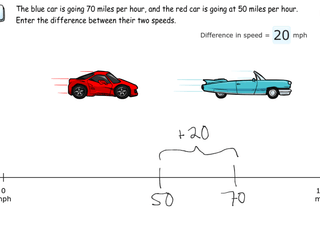# Interactive Problem Bank

•Fraction Circles
•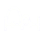Number Lines
•Fraction Bars
•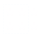Sets
•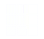Area Models
•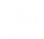Base Ten Blocks

## Ordering & Absolute Value

• Students make connections between positive and negative numbers and their distances from zero.
• Students order postive and negative numbers based on their relative position on the number line.

### Connecting with Integers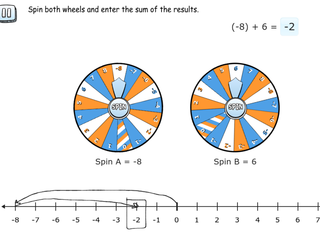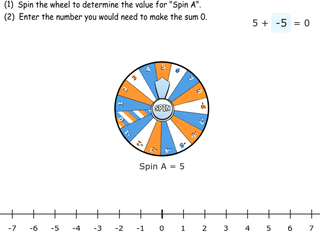### Ordering Integers on a Number Line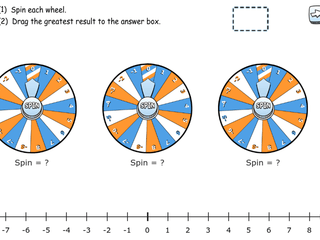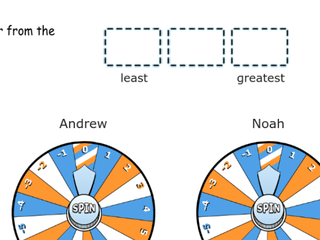### Exploring Inequality Statements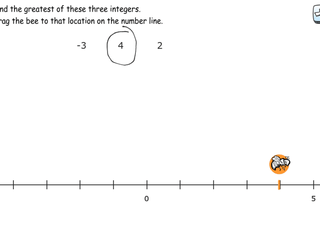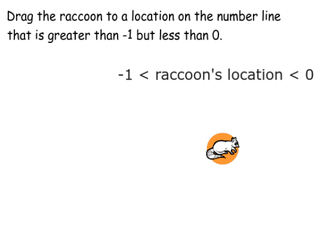### Estimating on a Vertical Number Line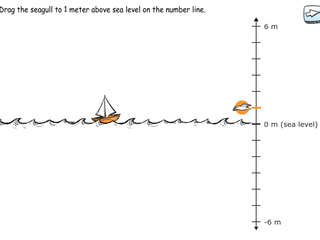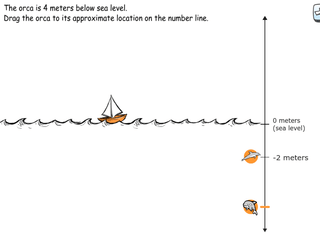### Modeling Distance on a Number Line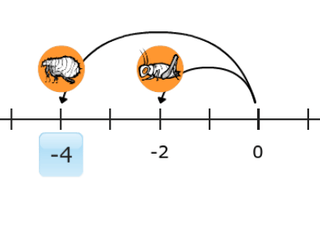### Finding Distances from Zero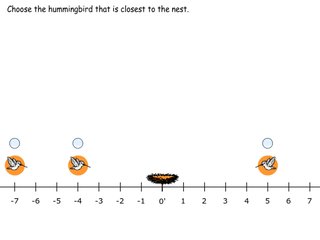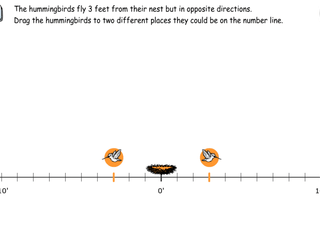### Understanding Absolute Value as Distance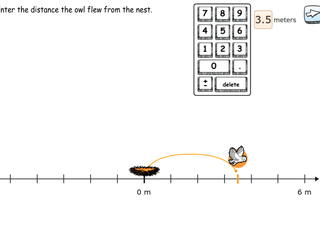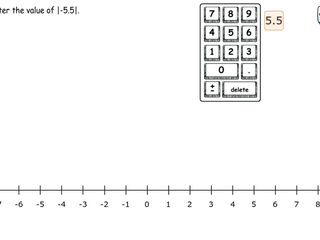### Making Sense of Absolute Value Notation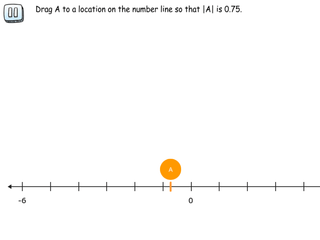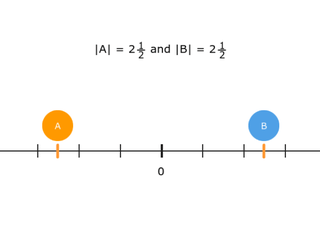### Absolute Value as a Distance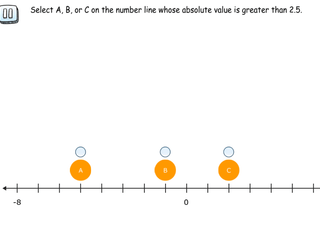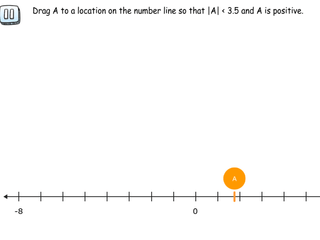### Finding Differences